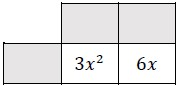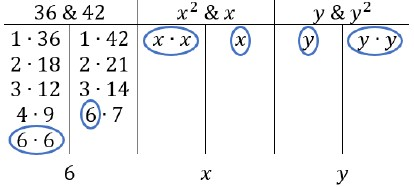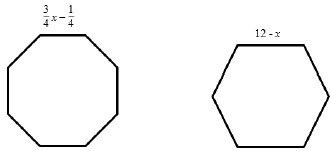# MA.8.AR.1.3Export Print
Rewrite the sum of two algebraic expressions having a common monomial factor as a common factor multiplied by the sum of two algebraic expressions.

### Examples

The expression 99x-11x³ can be rewritten as 11x(9-x²) or as -11x(-9+x² ).
General Information
Subject Area: Mathematics (B.E.S.T.)
Strand: Algebraic Reasoning
Status: State Board Approved

## Benchmark Instructional Guide

• Monomial

### Vertical Alignment

Previous Benchmarks

Next Benchmarks

### Purpose and Instructional Strategies

In grade 6, students learned to write the sum of two composite whole numbers that have a common factor, as a common factor multiplied by the sum of two whole numbers. In grade 7, students applied the properties of operations to add and subtract linear expressions with rational coefficients and determine if two linear expressions are equivalent. In grade 8, students rewrite the sum of two algebraic expressions having a common monomial factor as a common factor multiplied by the sum of two algebraic expressions. In Algebra 1, students will extend this learning to divide a polynomial by a monomial and to rewrite a polynomial expression as a product of polynomials by using a common factor.
• This benchmark is a foundational benchmark for work with the distributive property and factoring in more complex problems.
• Instruction begins with whole number coefficients to ensure students understand the process first. Then, move to rational number values (MTR.3.1).
• Instruction includes a review of common factors of two numbers (MA.6.NSO.3.2) so students can show understanding of the first step before applying it to the work of this benchmark.
• Instruction includes a review of the order of operations when working with multi-step problems (MTR.3.1).
• Instruction includes having expressions with more than one variable.
• For example, 24$x$$y$² + 8$x$$y$ can be rewritten as 8$x$$y$(3$y$ + 1).
• Emphasize properties of operations to determine equivalence when rewriting the expressions. Use manipulatives such as algebra tiles to represent the distributive property of multiplication over addition (MTR.2.1).
• Manipulatives
3$x$² + 6$x$• Area Models
3$x$² + 6$x$### Common Misconceptions or Errors

• Students may incorrectly apply the rules of integer arithmetic.
• Students may incorrectly apply the Laws of Exponents.
• Students may incorrectly change the degree of a variable in order to simplify terms.
• Students may incorrectly factor out only one of the terms from the parentheses.
• For example, in 18$x$$y$³ + 3$x$$y$², students may incorrectly factor it to 3$x$$y$²(6$y$ + 3$x$$y$²).

### Strategies to Support Tiered Instruction

• When factoring algebraic expressions, the teacher will provide instruction to review the process for finding greatest common factor (GCF) of two numbers before attempting to factor algebraic expressions. Once students understand the process of finding factors, more specifically the GCF, the teacher will provide instruction on factoring variables with and without exponents.
• Teacher models how to expand the degrees of variables to better understand what they truly have in common.
• For example, if the expression was 5$x$4$y$ + 10$x$²$y$³, it would be expanded as 5$x$$x$$x$$x$$y$ + 10$x$$x$$y$$y$$y$. Now that the expression is expanded, students can identify what variables each term has in common. This example shares two $x$’s and one $y$. Knowing this, students are able to find the GCF of 5 & 10, and finish factoring the expression.
• Instruction includes breaking up expressions to compare similar portions will help students focus on each step of factoring the algebraic expressions.
• For example, when factoring 36$x$²$y$ + 42$x$$y$², the expression should be broken up to factor as 36 & 42, $x$² & $x$, $y$ & $y$².• Once students understand how to factor each portion of the expression individually, teachers model how to “remove” the common factor from each portion of the expression, and how to use what is left over.

Tammy is designing seating around a new fire pit for her outdoor patio. One of the fire pit designs is a regular octagon with a side length of $\frac{\text{3}}{\text{4}}$$x$$\frac{\text{1}}{\text{4}}$. The other design is a regular hexagon with a side length of 12 − $x$.• Part A. Find the perimeter of the regular octagon fire pit.
• Part B. Find the perimeter of the regular hexagon fire pit.
• Part C. Write an expression with the fewest number of terms to show the difference between the perimeters of the two fire pits.
• Part D. Rewrite an equivalent expression from Part C as a common factor multiplied by the sum of two algebraic expressions.

### Instructional Items

Instructional Item 1
For each expression shown, determine an equivalent expression written as a common factor multiplied by the sum of two algebraic expressions.
a. 125$x$² + 15$x$
b. −24 − 36$y$³
c. $\frac{\text{3}}{\text{4}}$$x$$y$$\frac{\text{1}}{\text{4}}$ $x$²$y$³

*The strategies, tasks and items included in the B1G-M are examples and should not be considered comprehensive.

## Related Courses

This benchmark is part of these courses.
1200400: Foundational Skills in Mathematics 9-12 (Specifically in versions: 2014 - 2015, 2015 - 2022, 2022 and beyond (current))
1205050: M/J Accelerated Mathematics Grade 7 (Specifically in versions: 2014 - 2015, 2015 - 2020, 2020 - 2022, 2022 and beyond (current))
1205070: M/J Grade 8 Pre-Algebra (Specifically in versions: 2014 - 2015, 2015 - 2022, 2022 and beyond (current))
1204000: M/J Foundational Skills in Mathematics 6-8 (Specifically in versions: 2014 - 2015, 2015 - 2022, 2022 and beyond (current))
7812030: Access M/J Grade 8 Pre-Algebra (Specifically in versions: 2014 - 2015, 2015 - 2018, 2018 - 2019, 2019 - 2022, 2022 and beyond (current))

## Related Access Points

Alternate version of this benchmark for students with significant cognitive disabilities.
MA.8.AR.1.AP.3: Rewrite the sum of two linear algebraic expressions having a common whole number monomial factor as the common factor multiplied by the sum of two linear algebraic expressions.

## Related Resources

Vetted resources educators can use to teach the concepts and skills in this benchmark.

## Formative Assessment

Factored Forms:

Students are given two expressions and asked to rewrite each in factored form using the fewest number of terms.

Type: Formative Assessment

## Tutorial

Factor a Linear Expression by Taking a Common Factor:

This video demonstrates how to factor a linear expression by taking a common factor.

Type: Tutorial

## MFAS Formative Assessments

Factored Forms:

Students are given two expressions and asked to rewrite each in factored form using the fewest number of terms.

## Student Resources

Vetted resources students can use to learn the concepts and skills in this benchmark.

## Tutorial

Factor a Linear Expression by Taking a Common Factor:

This video demonstrates how to factor a linear expression by taking a common factor.

Type: Tutorial

## Parent Resources

Vetted resources caregivers can use to help students learn the concepts and skills in this benchmark.